Download Presentation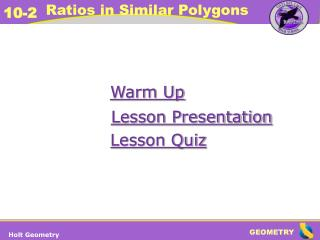Holt Geometry

# Holt Geometry - PowerPoint PPT Presentation

Warm Up. Lesson Presentation. Lesson Quiz. Holt Geometry.  Q  Z;  R  Y;  S  X; QR  ZY ; RS  YX ; QS  ZX. Warm Up 1. If ∆ QRS  ∆ ZYX , identify the pairs of congruent angles and the pairs of congruent sides. Solve each proportion. 2. 3. x = 9. x = 18.I am the owner, or an agent authorized to act on behalf of the owner, of the copyrighted work described.
Download Presentation## Holt Geometry

An Image/Link below is provided (as is) to download presentation

Download Policy: Content on the Website is provided to you AS IS for your information and personal use and may not be sold / licensed / shared on other websites without getting consent from its author.While downloading, if for some reason you are not able to download a presentation, the publisher may have deleted the file from their server.

- - - - - - - - - - - - - - - - - - - - - - - - - - E N D - - - - - - - - - - - - - - - - - - - - - - - - - -
Presentation TranscriptWarm Up

Lesson Presentation

Lesson Quiz

Holt Geometry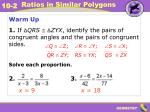Q  Z; R  Y; S  X;

QR  ZY; RS  YX; QS  ZX

Warm Up

1.If ∆QRS  ∆ZYX, identify the pairs of congruent angles and the pairs of congruent sides.

Solve each proportion.

2.3.

x = 9

x = 18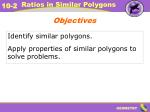Objectives

Identify similar polygons.

Apply properties of similar polygons to solve problems.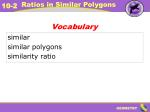Vocabulary

similar

similar polygons

similarity ratio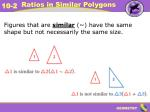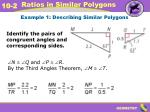Example 1: Describing Similar Polygons

Identify the pairs of congruent angles and corresponding sides.

0.5

N  Q and P  R.

By the Third Angles Theorem, M  T.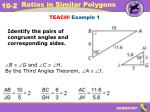TEACH! Example 1

Identify the pairs of congruent angles and corresponding sides.

B  G and C  H.

By the Third Angles Theorem, A  J.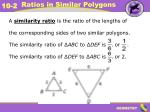A similarity ratiois the ratio of the lengths of

the corresponding sides of two similar polygons.

The similarity ratio of ∆ABC to ∆DEF is , or .

The similarity ratio of ∆DEF to ∆ABC is , or 2.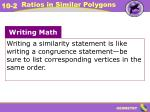Writing Math

Writing a similarity statement is like writing a congruence statement—be sure to list corresponding vertices in the same order.Example 2: Identifying Similar Polygons

Determine whether the polygons are similar. If so, write the similarity ratio and a similarity statement.

rectangles ABCD and EFGHExample 2 Continued

Step 1 Identify pairs of congruent angles.

A  E, B  F,

C  G, and D  H.

All s of a rect. are rt. s and are .

Step 2 Compare corresponding sides.TEACH! Example 2

Determine if ∆JLM ~∆NPS. If so, write the similarity ratio and a similarity statement.

Step 1 Identify pairs of congruent angles.

N  M, L  P, S  JThus the similarity ratio is , and ∆LMJ ~ ∆PNS.

TEACH! Example 2 Continued

Step 2 Compare corresponding sides.Example 3: Identifying Similar Polygons

Determine whether the polygons are similar. If so, write the similarity ratio and a similarity statement.

∆ABCD and ∆EFGHExample 3 Continued

Step 1 Identify pairs of congruent angles.

P  R and S  W

isos. ∆

Step 2 Compare corresponding angles.

mW = mS = 62°

mT = 180° – 2(62°) = 56°

Since no pairs of angles are congruent, the triangles are not similar.When you work with proportions, be sure the ratios compare corresponding measures.Example 4: Hobby Application

Find the length of the model to the nearest tenth of a centimeter.

Let x be the length of the model in centimeters. The rectangular model of the racing car is similar to the rectangular racing car, so the corresponding lengths are proportional.Example 4 Continued

5(6.3) = x(1.8)

Cross Products Prop.

31.5 = 1.8x

Simplify.

17.5 = x

Divide both sides by 1.8.

The length of the model is 17.5 centimeters.TEACH! Example 4

A boxcar has the dimensions shown.

A model of the boxcar is 1.25 in. wide. Find the length of the model to the nearest inch.TEACH! Example 4 Continued

1.25(36.25) = x(9)

Cross Products Prop.

45.3 = 9x

Simplify.

5 x

Divide both sides by 9.

The length of the model is approximately 5 inches.Lesson Quiz: Part I

1. Determine whether the polygons are similar. If so, write the similarity ratio and a similarity statement.

2. The ratio of a model sailboat’s dimensions to the actual boat’s dimensions is . If the length of the model is 10 inches, what is the length of the actual sailboat in feet?

no

25 ftLesson Quiz: Part II

3. Tell whether the following statement is sometimes, always, or never true. Two equilateral triangles are similar.

Always